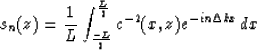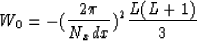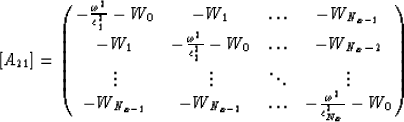Next: Tal-Ezer Method Up: THE GENERALIZED PHASE-SHIFT METHOD Previous: Horizontally Uniform Structures

## Arbitrary velocity structures

When the velocity structure varies in the lateral direction, the Fourier transform of velocity is no longer a delta function and the submatrix A21 has the form(15)
whereAnother derivation of submatrix A21 is possible in space frequency domain (Kosloff and Kessler, 1987). Whether the derivative in equation (6) is calculated by the Fourier method as with the ordinary phase-shift method (Gazdag, 1978), or by finite differences with periodic horizontal boundary conditions, it can be written as the cyclic convolution(16)
where Wi denotes a convolution operator. For example, for second-order finite differences,and, and Wi=0 for |i|>1. For Fourier second-derivative operator,andwith Nx=2L+1(Kosloff and Kessler, 1987). With the spatial discretization and specification of the second-derivative approximation, the elements of the Nx by Nx submatrix A21 are given by(17)
where ci=c(idx,z)Next: Tal-Ezer Method Up: THE GENERALIZED PHASE-SHIFT METHOD Previous: Horizontally Uniform Structures
Stanford Exploration Project
1/13/1998